# Rectangular Coordinates

//Rectangular Coordinates

## Coordinate Plane

The coordinate plane is used to represent ordered pairs of real numbers by points in a plane and determine relationships between those points and the segments, lines or curves that connect them.

The axes form four quadrants. The x values are positive from the origin to the right and are negative from the origin and to the left. The y values are positive above the origin and are negative below the origin. The quadrants are named by roman numerals I – IV starting in the upper right where both x and y values are positive and moving in a counter clockwise fashion.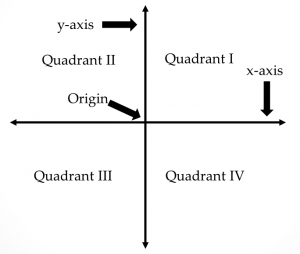One of the uses of a coordinate or Cartesian plane is to plot points to determine if there is a relationship between those points.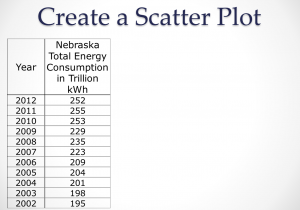You can also be asked to find the distance between two or more points plotted on a coordinate plane.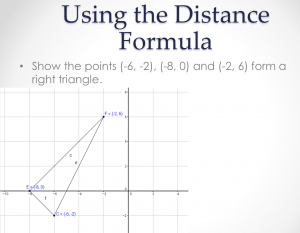Once you understand the relationship between the distance formula and the Pythagorean Theorem, you can use that relationship to prove that three points form a right triangle.

### Investigation – Loco for Loci

Desmos is an on-line graphing program that allows teachers to create activities. In the third screen of this activity by Dan Meyer, students drag a point to make it equal distance from two other points.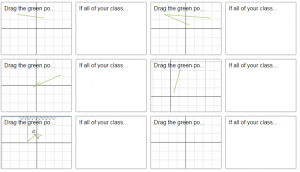The teacher’s version of the activity can show how all the points positioned by students are related to one another.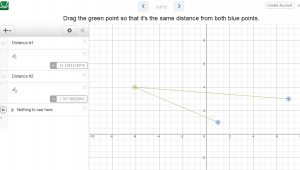This activity leads to one point that has a special relationship to two other points – the midpoint.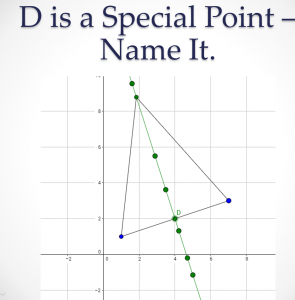From the investigation we can make the connection to the midpoint formula and even derive it if we desire.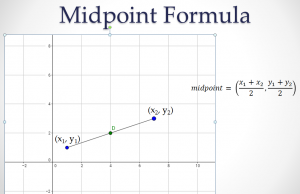Once you understand the midpoint formula, you can use it to solve problems like the one below.

Find the midpoint of the segment joining (-1, -2) and (3, 5).

By | 2017-11-13T22:02:01+00:00 August 16th, 2015|PreCalculus|0 Comments

### About the Author: Stacie Bender